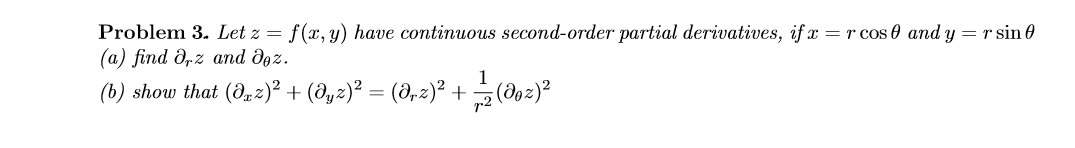# Problem 3. Let z = (а) find Ә,z and дөz. (b) show that (d,2)² + (@yz)² = (8,2)² + (do2)? f(x, y) have continuous second-order partial derivatives, if x = r cos 0 and y = r sin 0

Question

Let z = f(x,y) have continuous second-order partial derivatives, if x = rcosθ and y = rsinθ

(a) ﬁnd ∂rz and ∂θz.

(b) show that (∂xz)^2 + (∂yz)^2 = (∂rz)^2 + 1/r^2 (∂θz)^2.help_outlineImage TranscriptioncloseProblem 3. Let z = (а) find Ә,z and дөz. (b) show that (d,2)² + (@yz)² = (8,2)² + (do2)? f(x, y) have continuous second-order partial derivatives, if x = r cos 0 and y = r sin 0 fullscreen

### Want to see this answer and more?

Experts are waiting 24/7 to provide step-by-step solutions in as fast as 30 minutes!*

*Response times may vary by subject and question complexity. Median response time is 34 minutes for paid subscribers and may be longer for promotional offers.
Tagged in
Math
Calculus

### Applications of Derivative